## Using the test statistic

The test statistic is a standardized measure that allows us to calculate the probability of an event. Although the formulas appear to change in every section, the test statistic is almost always the number of standard deviations the statistic is from the mean. Here are four different examples that you probably learned in class.

1-sample means test (Z-test)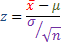1-proportion means test (Z-test)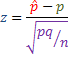2-sample means test (Z-test)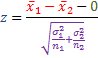2-sample means test (dependent samples)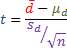Although the four equations look very different, they all have exactly the same structure: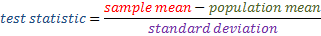The reason the formulas all look different is because we determine the population mean and standard deviation differently for different sample tests. But if you remember that all the test statistics have the same basic structure, you will find your calculations are a lot easier to figure out.

Statistics
Blue Taste Theme created by Jabox